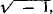# imaginary number

Also found in: Dictionary, Thesaurus, Medical, Legal, Wikipedia.
Related to imaginary number: pure imaginary number, complex number

## imaginary number:

see numbernumber,
entity describing the magnitude or position of a mathematical object or extensions of these concepts. The Natural Numbers

Cardinal numbers describe the size of a collection of objects; two such collections have the same (cardinal) number of objects if their
.

## Imaginary Number

a number of the form x + iy, where i =x and y are real numbers and y ≠ 0; that is, a complex number that is not real. Imaginary numbers of the form iy are called pure imaginary; sometimes only the latter are referred to as imaginary numbers. The term “imaginary number” appeared after such numbers had already entered general use although their real meaning had not been ascertained.

## imaginary number

[ə′maj·ə‚ner·ē ′nəm·bər]
(mathematics)
A complex number of the form a + bi, with b not equal to zero, where a and b are real numbers, and i = √(-1); some mathematicians require also that a = 0. Also known as imaginary quantity.
References in periodicals archive ?
If another axis is added, a vertical (imaginary) axis at x = 0, this will represent the imaginary numbers. Since i = [square root of -1], this also means that [i.sup.2] = -1.
In our paper the imaginary number raised to the fractional power leads to the exponentially decaying solution and hence leads to the correct limit when t [right arrow] [infinity], namely, zero total probability.
They were to select (a) a periodic, which included lithium, hydrogen, cesium, francium, sodium, rubidium, and potassium; (b) an isotope, which included kalium, oxygen, deuterium, and tritium; (c) an imaginary number, which included Jerion, Mark's Horse, or Bromius; (d) Mark Carscian, an adult human male and an official representative of the Mathematician; and (e) the Mathematician, who is the ruler of the Land of Mathematics and was described as a tiny, kind-hearted, white-bearded man who wore a simple crown.
He incorrectly states that imaginary numbers were introduced historically to be solutions to equations, rather than a means to a real solution.
Similar to the one rogue wave, if the pair of [m.sub.1], [m.sub.2] are imaginary numbers and [m.sub.3], [m.sub.4] are real numbers or the opposite, the exciting interaction between lump solution and line rogue wave will appear, which is shown in Figure 8.
Kristina first drew from literacy-related principles found in the text, then connected this information on activating prior knowledge to promote content area comprehension to an online article that she had found (independently) on imaginary numbers (Azad, 2013), using the principle of prior knowledge in relating imaginary numbers to negative numbers:
They also assume a high-school level of mathematics--trigonometry, matrix algebra, complex and imaginary numbers, and some calculus.
Now, except for mathematicians and scientists who learn discursive ways as stepping stones to bending reality to irrational and imaginary numbers and the theories they germinate, it seems that most humans, propelled by almost universal access to micro- and macro-realities, are treating laws/boundaries as realities to be transcended--no longer real.
Businesses, employers have prejudices against unions." Imaginary numbersFollowing a new regulation introduced in 2009, which came into force in 2012, measurement of the membership percentages has started to be conducted by the Social Security Institution (SGK) instead of the data provided by unions and the threshold for collective bargaining was lowered from 10, a much criticized level by the UN's International Labor Organization (ILO), to one percent.Previously in order for unions to be able to qualify, imaginary numbers were used, Celik said, since if real numbers were used only a handful unions could have qualified.
Input to the function is array of real numbers, but computing of imaginary numbers is also possible.
"Some sides gave imaginary numbers for revenues with estimates reaching \$1 billion or \$1.5 billion.
Clark's algebra test was in imaginary numbers. But there was nothing imaginary about the number Clark posted in the 1,000.

Site: Follow: Share:
Open / Close